# Disintegration Effects in Processing

Updated 2018-08-02

In this small project, my objective is to take an input image and disintegrate it into particles. I will be using Processing 3 because it’s easy to do quick graphical interactives and I’m familiar with it.

This is my first implementation and it is a basic and limited one. The image is loaded from file, and a grid of small rectangles are drawn such that it resembles the original image. Here is what it looks like (with black strokes around each rectangle):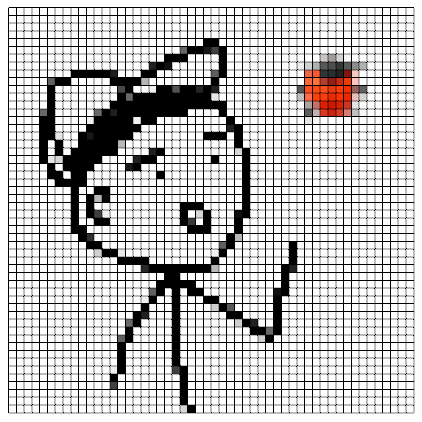Next, in the 3D space, I applied a transform for each rectangle in the z-axis direction. How much translation is a function of x and y. In this case, I used the exponential function $$e^{-kx^2}$$, where $$x$$ is the horizontal coordinate, and $$k$$ is some scaling parameter.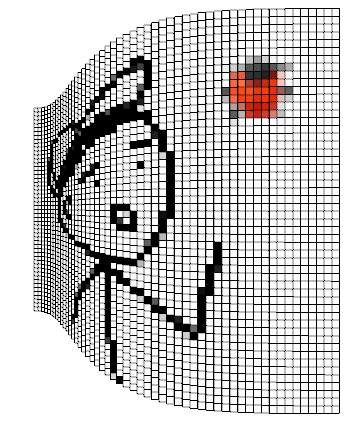But this looks unnatural, so I made the output of the exponential function define the amplitude of a Perlin noise, a function of x and y. Along with that, I made it so the more the rectangles gets translated, the smaller it shrinks to give off the decaying effect.

Finally, removing the strokes and decreasing the rectangle size (to 1px) yield some better effect: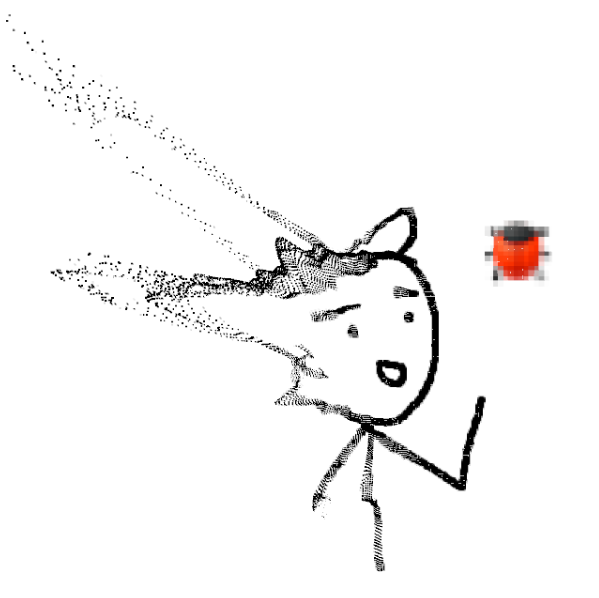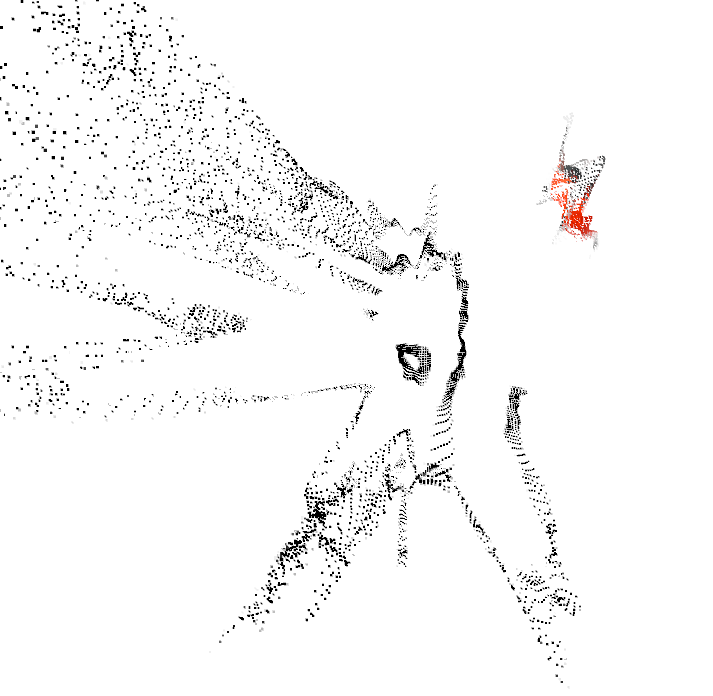Remember that parameter $$k$$ we put on to the transform function? Well we can animate it. I made it start off at 1.0, then each frame multiply it by 90%. This gives off of a slowly disintegrating effect. I also added a 3D interactive camera using PeasyCam library so that I can easily move the camera around. Here are the results: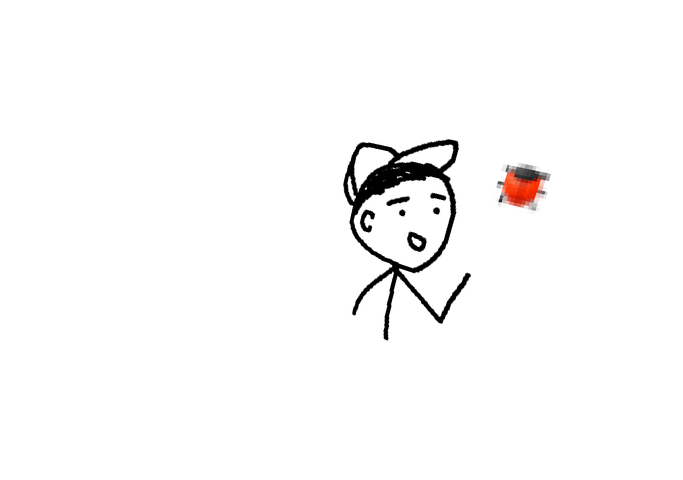The source code can be found here.